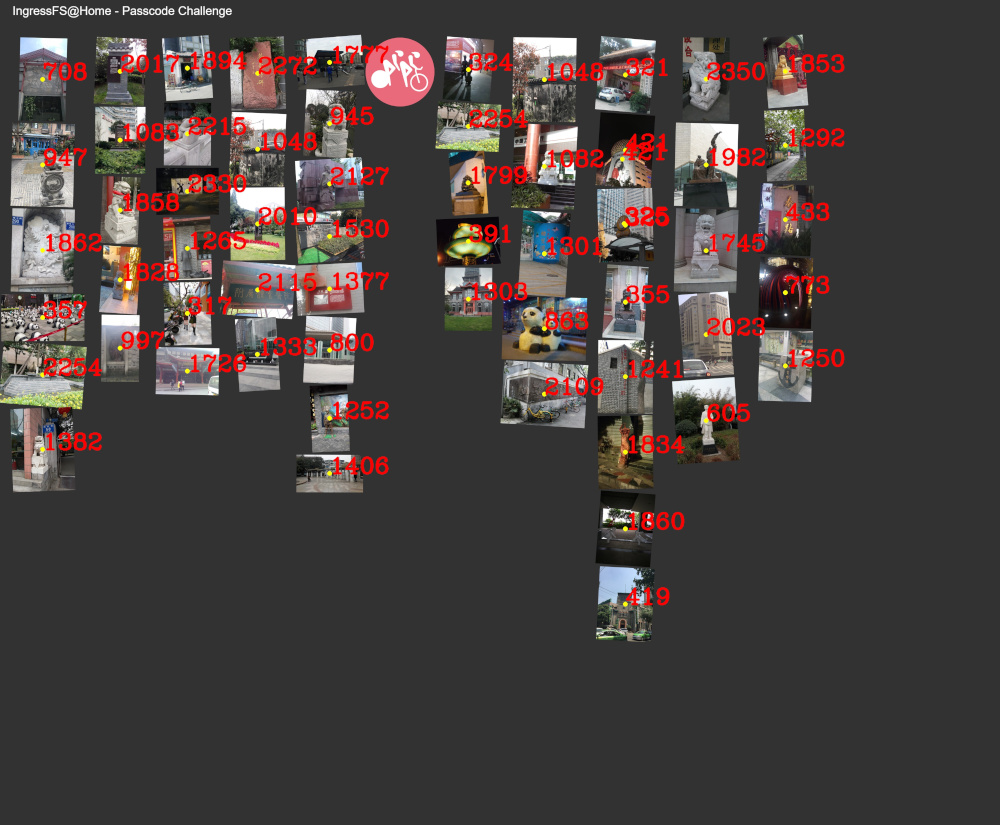## 生成网格

$$M= \begin{pmatrix} \displaylines{ (P_{x1}, P_{y1}) & \cdots & (P_{xi}, P_{y1}) \\ \vdots & \ddots & \vdots \\ (P_{x1}, P_{yj}) & \cdots & (P_{xi}, P_{yj}) } \end{pmatrix}$$

$$\underset {\mathbf {Col}}{\operatorname {arg,min}} \sum_{i=1}^k\sum_{P \in Col_i}\left|{\mathbf P_x}-\mu_{i}\right|^{2}$$

## 错误识别

• 有同一张照片的不同部分被识别为了相同的结果。
• 有同一张照片的不同部分被识别为了不同的结果。